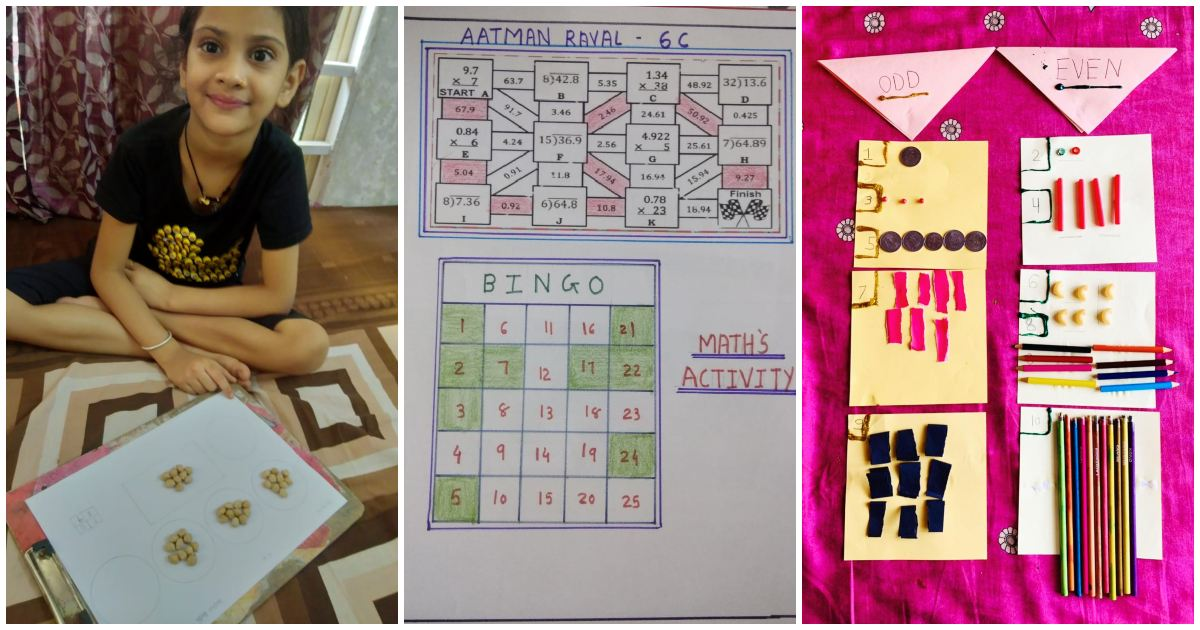GLOBAL SCHOOL NEWS
April 26, 2021

## Students experience the world of Math–e-magic during the Math Camp held at GIIS Ahmedabad Students experience the world of Math–e-magic during the Math Camp held at GIIS AhmedabadWhy do children dread Mathematics? Because of the wrong approach. Because it is looked at as a subject.

— Shakuntala Devi, well known Indian writer and Mental Calculator

If we go down deep enough into anything, we will find Mathematics. Mathematics is not only about numbers, equations, computations, or algorithms: it is about understanding. Everything around us is Mathematics. Everything around us is numbers. Galileo Galilei, the Italian physicist, and astronomer was not wrong when he said that even Nature is written in mathematical language.

There is nothing such as boring Mathematics. However, the children often dread Math because it is approached as a subject. But the chief end of mathematical study must be to make the students think. Then, the students will find Math interesting and realize that it can be fun.

In this context, a special Math Camp was conducted at GIIS Ahmedabad on 23rd and 24th April 2021 for the students of Grades 1 to 10 to hone their Mathematical skills.

Based on the performance analysis of the students in the Math Proficiency Test held earlier, various stimulating activities were carefully designed by the teachers to plug in their learning gaps in Math.

A Fun-filled Scoop and Group activity was conducted for Grade 1 to reinforce the concept of Place Value. The objective of this activity was to help the students to learn about easy counting and comparing the numbers in ascending and descending order. An interesting pairing and coloring activity built around the concept of odd and even numbers was organized for the students of Grade 2. Activities based on Place Value, Multiplication Table, and Mental Math Quiz were held for the students of Grade 3. Special emphasis was given to enhance the learning of the four operations –Add, Subtract, Multiply and Divide through Picture Puzzle and Dice activity in Grade 4. A Quiz was also conducted on the Quizziz platform based on Mental Math and logical reasoning questions. The students of Grade 5 participated in interesting activities related to finding area and perimeter through GeoBoards and decimals with base ten blocks which were followed by an exciting Maths Quiz.

The students of Grade 6 had great fun solving the HCF Bingo, solving sums based on multiplication and division with decimals to find and color the designated path, solving the puzzles on LCM and Fractions as well as participating in Mental Math Quiz. Whereas, the Grade 7 students had crossword based on algebraic expressions, activity for using mensuration to find the perimeter or irregular figures, decoding message using addition and subtraction of integers, solving equations to draw the path from start to finish, and Mental Math Quiz. The students of Grade 8 had activities to solve linear equations, evaluate exponents, simplify algebraic equations and solve equations to draw the path from start to finish along with Math Quiz.

Verifying Pythagoras theorem using Bhaskara’s method, finding proof of algebraic identity in 3D model, calculating the change in the surface area and the volume of the solid when it is converted into different solid or multiple solids, either of the same or different shapes, Math Tambola and Quiz based on ‘Who Wants to be a Millionaire’ format were some interesting activities conducted for Grades 9 and 10.

Mr. Caesar D’silva, Principal, GIIS Ahmedabad said, “The activities conducted during the Math Camp were designed to encourage creativity, problem-solving, collaborative skills, communication skills among the students as well as to engage them in real-world data. We are really happy that the students enjoyed participating in the Maths Camp and the enthusiasm for learning the subject has reached a new high.”

The students' involvement was remarkable.

• 0